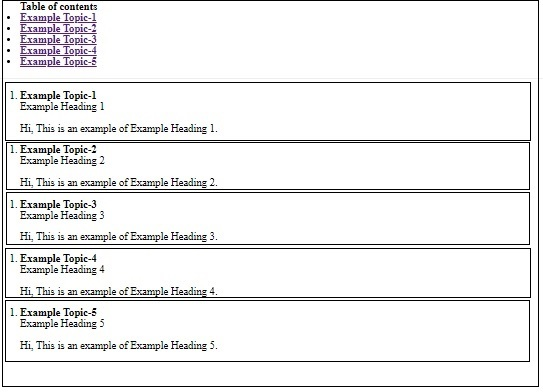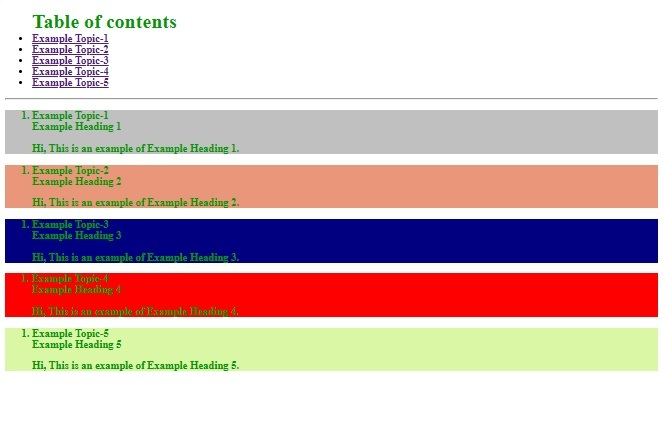# How div class and id is useful in HTML ?

In this article, we will discuss how the id, div, and class is useful in HTML. Id, Div, and class are important concepts of structuring the HTML page. let’s discuss one by one.

Now, consider a use case where you want to implement a table of contents of any book and want to go directly to the topics which you want to read. In this case, you can implement the Id attribute in HTML5 and can link all the topics with a table of contents. Now, you can directly click and can directly go to the topics which you want to read.

 ` ` `<``html``> ` ` `  `<``head``> ` `    ``<``title``>Id Div and Class ` ` ` ` `  `<``body``> ` `    ``<``div``> ` `        ``<``ul``> ` `            ``<``div``> ` `                ``<``strong``>Table of contents ` `                ``<``li``> ` `                    ``<``a` `href``=``"#T1"``> ` `                        ``<``strong``> ` `                            ``Example Topic-1 ` `                        `` ` `                    `` ` `                `` ` ` `  `                ``<``li``> ` `                    ``<``a` `href``=``"#T2"``> ` `                        ``<``strong``> ` `                            ``Example Topic-2 ` `                        `` ` `                    `` ` `                `` ` ` `  `                ``<``li``> ` `                    ``<``a` `href``=``"#T3"``> ` `                        ``<``strong``> ` `                            ``Example Topic-3 ` `                        `` ` `                    `` ` `                `` ` ` `  `                ``<``li``> ` `                    ``<``a` `href``=``"#T4"``> ` `                        ``<``strong``> ` `                            ``Example Topic-4 ` `                        `` ` `                    `` ` `                `` ` ` `  `                ``<``li``> ` `                    ``<``a` `href``=``"#T5"``> ` `                        ``<``strong``> ` `                            ``Example Topic-5 ` `                        `` ` `                    `` ` `                `` ` `            `` ` `        `` ` `        ``<``hr` `/> ` ` `  `        ``<``div` `id``=``"T1"``> ` `            ``<``ol``> ` `                ``<``li``> ` `                    ``<``strong``>Example Topic-1 ` `                    ``<``br` `/> ` `                    ``Example Heading 1 ` `                    ``<``p``> ` `                        ``Hi, This is an example of  ` `                        ``Example Heading 1. ` `                    `` ` `                `` ` `            `` ` `        `` ` ` `  `        ``<``div` `id``=``"T2"``> ` `            ``<``ol``> ` `                ``<``li``> ` `                    ``<``strong``>Example Topic-2 ` `                    ``<``br` `/> ` `                    ``Example Heading 2 ` `                    ``<``p``> ` `                        ``Hi, This is an example of  ` `                        ``Example Heading 2. ` `                    `` ` `                `` ` `            `` ` `        `` ` ` `  `        ``<``div` `id``=``"T3"``> ` `            ``<``ol``> ` `                ``<``li``> ` `                    ``<``strong``>Example Topic-3 ` `                    ``<``br` `/> ` `                    ``Example Heading 3 ` `                    ``<``p``> ` `                        ``Hi, This is an example of  ` `                        ``Example Heading 3. ` `                    `` ` `                `` ` `            `` ` `        `` ` ` `  `        ``<``div` `id``=``"T4"``> ` `            ``<``ol``> ` `                ``<``li``> ` `                    ``<``strong``>Example Topic-4 ` `                    ``<``br` `/> ` `                    ``Example Heading 4 ` `                    ``<``p``> ` `                        ``Hi, This is an example of  ` `                        ``Example Heading 4. ` `                    `` ` `                `` ` `            `` ` `        `` ` ` `  `        ``<``div` `id``=``"T5"``> ` `            ``<``ol``> ` `                ``<``li``> ` `                    ``<``strong``>Example Topic-5 ` `                    ``<``br` `/> ` `                    ``Example Heading 5 ` `                    ``<``p``> ` `                        ``Hi, This is an example of  ` `                        ``Example Heading 5. ` `                    `` ` `                `` ` `            `` ` `        `` ` `    `` ` ` ` ` `  ` `

You can verify the results by executing the above code. Now, let’s see the output with the page structure.
Output:In the above output screen, you can directly see how Id and Div works, and also you can see that how Div is useful to structure your webpage.

Now, here you will see how you can add more functionality to your webpage by using CSS with the help of Id and Div and class.

 ` ` `<``html``> ` ` `  `<``head``> ` `    ``<``title``>Id Div and Class ` ` `  `    ``<``style``> ` `        ``#TOC { ` `            ``background-color: white; ` `            ``color: #009900; ` `            ``font-size: 30px; ` `            ``font-weight: bold; ` `            ``text-align: left; ` `        ``} ` ` `  `        ``.T1 { ` `            ``background-color: SILVER; ` `            ``color: #009900; ` `            ``font-weight: bold; ` `        ``} ` ` `  `        ``.T2 { ` `            ``background-color: DARKSALMON; ` `            ``color: #009900; ` `            ``font-weight: bold; ` `        ``} ` ` `  `        ``.T3 { ` `            ``background-color: NAVY; ` `            ``color: #009900; ` `            ``font-weight: bold; ` `        ``} ` ` `  `        ``.T4 { ` `            ``background-color: red; ` `            ``color: #009900; ` `            ``font-weight: bold; ` `        ``} ` ` `  `        ``.T5 { ` `            ``background-color: #daf7a6; ` `            ``color: #009900; ` `            ``font-weight: bold; ` `        ``} ` `    `` ` ` ` ` `  `<``body``> ` `    ``<``div``> ` `        ``<``div``> ` `            ``<``ul``> ` `                ``<``div` `id``=``"TOC"``> ` `                    ``<``strong``>Table of contents ` `                `` ` `                ``<``li``> ` `                    ``<``a` `href``=``"#T1"``><``strong``> ` `                            ``Example Topic-1 ` `                `` ` `                ``<``li``> ` `                    ``<``a` `href``=``"#T2"``><``strong``> ` `                            ``Example Topic-2 ` `                `` ` `                ``<``li``> ` `                    ``<``a` `href``=``"#T3"``><``strong``> ` `                            ``Example Topic-3 ` `                `` ` `                ``<``li``> ` `                    ``<``a` `href``=``"#T4"``><``strong``> ` `                            ``Example Topic-4 ` `                `` ` `                ``<``li``> ` `                    ``<``a` `href``=``"#T5"``><``strong``> ` `                            ``Example Topic-5 ` `                `` ` `            `` ` `            ``<``hr` `/> ` ` `  `            ``<``div` `class``=``"T1"``> ` `                ``<``div` `id``=``"T1"``> ` `                    ``<``ol``> ` `                        ``<``li``> ` `                            ``<``strong``> ` `                                ``Example Topic-1 ` `                            ``<``br` `/> ` `                            ``Example Heading 1 ` `                            ``<``p``> ` `                                ``Hi, This is an example of  ` `                                ``Example Heading 1. ` `                            `` ` `                        `` ` `                    `` ` `                `` ` `            `` ` ` `  `            ``<``div` `class``=``"T2"``> ` `                ``<``div` `id``=``"T2"``> ` `                    ``<``ol``> ` `                        ``<``li``> ` `                            ``<``strong``>Example Topic-2 ` `                            ``<``br` `/> ` `                            ``Example Heading 2 ` `                            ``<``p``> ` `                                ``Hi, This is an example of  ` `                                ``Example Heading 2. ` `                            `` ` `                        `` ` `                    `` ` `                `` ` `            `` ` ` `  `            ``<``div` `class``=``"T3"``> ` `                ``<``div` `id``=``"T3"``> ` `                    ``<``ol``> ` `                        ``<``li``> ` `                            ``<``strong``>Example Topic-3 ` `                            ``<``br` `/> ` `                            ``Example Heading 3 ` `                            ``<``p``> ` `                                ``Hi, This is an example of  ` `                                ``Example Heading 3. ` `                            `` ` `                        `` ` `                    `` ` `                `` ` `            `` ` ` `  `            ``<``div` `class``=``"T4"``> ` `                ``<``div` `id``=``"T4"``> ` `                    ``<``ol``> ` `                        ``<``li``> ` `                            ``<``strong``>Example Topic-4 ` `                            ``<``br` `/> ` `                            ``Example Heading 4 ` `                            ``<``p``> ` `                                ``Hi, This is an example of  ` `                                ``Example Heading 4. ` `                            `` ` `                        `` ` `                    `` ` `                `` ` `            `` ` ` `  `            ``<``div` `class``=``"T5"``> ` `                ``<``div` `id``=``"T5"``> ` `                    ``<``ol``> ` `                        ``<``li``> ` `                            ``<``strong``>Example Topic-5 ` `                            ``<``br` `/> ` `                            ``Example Heading 5 ` `                            ``<``p``> ` `                                ``Hi, This is an example of  ` `                                ``Example Heading 5. ` `                            `` ` `                        `` ` `                    `` ` `                `` ` `            `` ` `        `` ` `    `` ` ` ` ` `  ` `

You can verify the results by executing the above code. Now, let’s see the output with the page structure.
Output:In the above example, you can directly see that by using Id we can add individual functionality to different headings, and class is for common we can write the CSS script once and can use it anywhere and it is also useful for reusability.My Personal Notes arrow_drop_upCheck out this Author's contributed articles.

If you like GeeksforGeeks and would like to contribute, you can also write an article using contribute.geeksforgeeks.org or mail your article to contribute@geeksforgeeks.org. See your article appearing on the GeeksforGeeks main page and help other Geeks.

Please Improve this article if you find anything incorrect by clicking on the "Improve Article" button below.